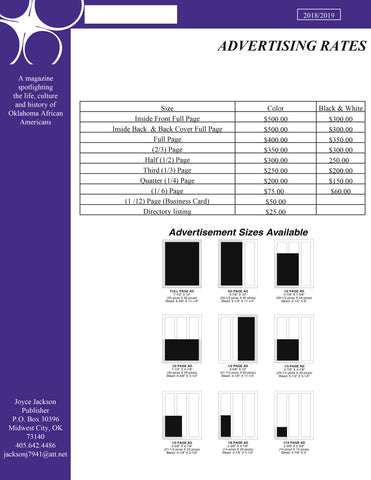Below you will find documents pertaining to the editorial schedule, advertising rates and guidelines.SHADES of Oklahoma Advertising Rates by Frequency  (Prices in effect until  12/31/18)

Full page          X 1    = \$400

Full page         X 2     = \$700   (\$350 each)

Full page         X 3     = \$900 (\$300 each)

Full page         X 4     = \$1,000 (\$250 each)

Half page      X 1   = \$300

Half page      X 2   = \$500 (\$250 each)

Half page      X 3   = \$600 (\$200 each)

Half page      X 4   = \$700 (\$175 each)

Quarter page  X 1  = \$200

Quarter page  X 2  = \$350 (\$175 each)

Quarter page  X 3  = \$450  (\$150 each)

Quarter page  X 4  =  \$500 ( \$125 each)